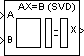# SVD Solver

Solve AX=B using singular value decomposition

## Library

Math Functions / Matrices and Linear Algebra / Linear System Solvers

`dspsolvers`

•## Description

The SVD Solver block solves the linear system AX=B, which can be overdetermined, underdetermined, or exactly determined. The system is solved by applying singular value decomposition (SVD) factorization to the M-by-N matrix A, at the A port. The input to the B port is the right side M-by-L matrix, B. The block treats length-M unoriented vector input as an M-by-1 matrix.

The output at the X port is the N-by-L matrix, X. X is chosen to minimize the sum of the squares of the elements of B-AX (the residual). When B is a vector, this solution minimizes the vector 2-norm of the residual. When B is a matrix, this solution minimizes the matrix Frobenius norm of the residual. In this case, the columns of X are the solutions to the L corresponding systems AXk=Bk, where Bk is the kth column of B, and Xk is the kth column of X.

X is known as the minimum-norm-residual solution to AX=B. The minimum-norm-residual solution is unique for overdetermined and exactly determined linear systems, but it is not unique for underdetermined linear systems. Thus when the SVD Solver block is applied to an underdetermined system, the output X is chosen such that the number of nonzero entries in X is minimized.

## Parameters

Show error status port

Select to enable the E output port, which reports a failure to converge. The possible values you can receive on the port are:

• `0` — The singular value decomposition calculation converges.

• `1` — The singular value decomposition calculation does not converge.

If the singular value decomposition calculation fails to converge, the output at port X is an undefined matrix of the correct size.

## Supported Data Types

PortSupported Data Types

A

• Double-precision floating point

• Single-precision floating point

B

• Double-precision floating point

• Single-precision floating point

X

• Double-precision floating point

• Single-precision floating point

E

• Boolean

 Autocorrelation LPC DSP System Toolbox Cholesky Solver DSP System Toolbox LDL Solver DSP System Toolbox Levinson-Durbin DSP System Toolbox LU Inverse DSP System Toolbox Pseudoinverse DSP System Toolbox QR Solver DSP System Toolbox Singular Value Decomposition DSP System Toolbox

See Linear System Solvers for related information.

## Extended Capabilities

Introduced before R2006a

## SupportGet trial now